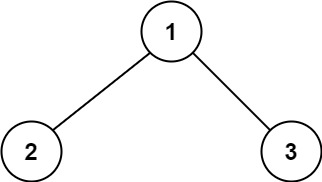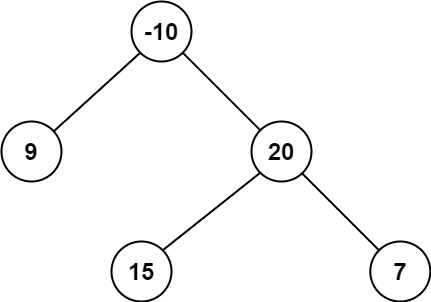# 124. Binary Tree Maximum Path Sum

Hard
A path in a binary tree is a sequence of nodes where each pair of adjacent nodes in the sequence has an edge connecting them. A node can only appear in the sequence at most once. Note that the path does not need to pass through the root.
The path sum of a path is the sum of the node's values in the path.
Given the `root` of a binary tree, return the maximum path sum of any non-empty path.
Example 1:Input: root = [1,2,3]
Output: 6
Explanation: The optimal path is 2 -> 1 -> 3 with a path sum of 2 + 1 + 3 = 6.
Example 2:Input: root = [-10,9,20,null,null,15,7]
Output: 42
Explanation: The optimal path is 15 -> 20 -> 7 with a path sum of 15 + 20 + 7 = 42.
Constraints:
• The number of nodes in the tree is in the range `[1, 3 * 10^4]`.
• `-1000 <= Node.val <= 1000`

### 解題

/**
* Definition for a binary tree node.
* type TreeNode struct {
* Val int
* Left *TreeNode
* Right *TreeNode
* }
*/
func maxPathSum(root *TreeNode) int {
ans := math.MinInt32
var helper func(*TreeNode)int
helper = func(root *TreeNode) int {
if root == nil {
return 0
}
left := max(helper(root.Left), 0)
right := max(helper(root.Right), 0)
ans = max(ans, left + right + root.Val)
return max(left, right) + root.Val
}
helper(root)
return ans
}
func max(a, b int) int {
if a > b {
return a
}
return b
}